##### Actions

(diff) ← Older revision | Latest revision (diff) | Newer revision → (diff)

Voting paradoxes describe counter-intuitive election outcomes. The mathematical study started in 1770 when J.C. Borda [a1] constructed an example to argue that the plurality vote (where each voter votes for one candidate) used to select members to the French Academy of Science was flawed. Borda's example hasvoters with the (complete, transitive) preferences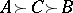,with, andwith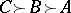. Here, the plurality ranking of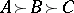conflicts with the pairwise rankings of,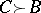,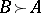with the respective tallies of,,. Borda suggested an alternative method, the Borda count, where withalternatives a voter'sth ranked candidate receivespoints,The Borda count outcome for the example is the more reasonablewith thetally.

More generally, ancandidate election can be tallied with a voting vector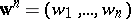where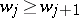,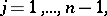and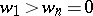. (The plurality vote corresponds to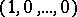while the Borda count is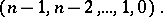) With the infinite number of ways to tally ballots, P.S. Laplace and other mathematicians started a two-century controversy by questioning whichwould be "optimal" . The difficulty of this area is partially captured by Arrow's assertion that no procedure does what is commonly expected (cf. Arrow impossibility theorem). See [a2], [a3] for a flavour of the rich history of this area and [a7] for a mathematical introduction.

What makes voting paradoxes difficult to analyze is that they are "counter-intuitive" , so it is not clear what to search for. Consequently, while several paradoxes have been discovered (e.g., see [a5]), they are limited. However, by using symmetry and arguments from chaotic dynamics, all possible voting paradoxes finally have been characterized [a6], [a8]. To describe them, notice thatalternatives define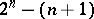subsets with two or more candidates; for each subset, choose a voting vector. D. Saari showed that for almost-all choices of voting vectors, "anything can happen" . Namely, for each of thesubsets, choose any ranking of the candidates. There exists an example of voters, each with a transitive ranking of the candidates, where when the ballots for each subset are tallied with the specified voting vector, the outcome is the selected one. More specifically, the vector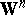, which designates thesechoices of voting vectors, is in an appropriate-dimensional vector space. There is a lower-dimensional algebraic setso that if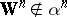, then this "anything can happen" conclusion holds. A positive assertion is that the number and kind of paradoxes is minimized if the Borda count is used to tally each subset of candidates. More precisely, if a listing of rankings (i.e., a voting paradox) can occur with the Borda count, it also occurs with any other. However, ifuses a non-Borda count choice to tally a subset of candidates, thenadmits paradoxes that never occur with the Borda count. As an illustration, only the Borda count rankings are related with the pairwise rankings; e.g., for any other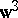there are voters' preferences where the election outcomes are the same as in the introductory example.
To illustrate the Borda count consistency advantage over other procedures, letand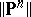denote, respectively, the number of lists of distinct election rankings allowed by the Borda count and the plurality vote over thesubsets. Even for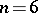, it turns out that. These results indicate that the Borda count is the pragmatic answer to Laplace's question about the optimal choice of a voting procedure.
A closely related assertion holds for rankings determined by non-parametric methods of statistics. In particular, D. Haunsperger [a4] published a similar theorem, showing that foralternatives, almost-all non-parametric methods allow anything to happen. She then showed that the Krushkal–Wallis test plays the role of the Borda count by minimizing the number and kinds of paradoxes that can occur.